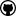org.dllearner.experiments

## Class ExMakerFixedSize

• ```public class ExMakerFixedSize
extends Object```
used to randomize examples and split them into training and test sets gets a fixed number of examples
Author:
Sebastian Hellmann
• ### Constructor Summary

Constructors
Constructor and Description
`ExMakerFixedSize(Examples examples)`
```ExMakerFixedSize(Examples examples, boolean randomize)```
• ### Method Summary

All Methods
Modifier and Type Method and Description
`static void` `main(String[] args)`
`static String` `pickOneRandomly(String[] from)`
`Examples` `select(int both)`
same as select(int,int) uses both times the same number
`Examples` ```select(int nrOfPos, int nrOfNeg)```
returns a new example object based on ALL (train and test) examples in the old set picks a fixed number of examples, puts them into training sets, rest to test set
• ### Methods inherited from class java.lang.Object

`equals, getClass, hashCode, notify, notifyAll, toString, wait, wait, wait`
• ### Constructor Detail

• #### ExMakerFixedSize

`public ExMakerFixedSize(Examples examples)`
• #### ExMakerFixedSize

```public ExMakerFixedSize(Examples examples,
boolean randomize)```
• ### Method Detail

• #### main

`public static void main(String[] args)`
• #### select

`public Examples select(int both)`
same as select(int,int) uses both times the same number
Parameters:
`both` -
Returns:
• #### select

```public Examples select(int nrOfPos,
int nrOfNeg)```
returns a new example object based on ALL (train and test) examples in the old set picks a fixed number of examples, puts them into training sets, rest to test set
Parameters:
`nrOfPos` -
`nrOfNeg` -
Returns:
• #### pickOneRandomly

`public static String pickOneRandomly(String[] from)`DL-Learner is licenced under the terms of the GNU General Public License.